PART 1: Set Theory

## 所有归纳集为什么不是集合

• 正则公理（Axiom of Regularity）$\forall A,\exists x:(\exists z:z\in A)\Rightarrow (x\in A\wedge (\neg\exists y: y\in A\wedge y\in x))$.

• 自然数属于所有归纳集的集合；
• 自然数集 $\mathbb{N}$包含于所有归纳集的集合.

## 基数和序数

• 序数是表示次序的数（数数的时候会数到的数）：$1,2,\ldots,n,\ldots,\aleph_0,\aleph_0+1,\ldots,\aleph_1,\ldots,\aleph_n,\ldots$
• 基数是序数的等价类：$1,2,\ldots,n,\ldots,\aleph_0,\aleph_1,\aleph_2,\ldots,\aleph_n,\ldots$

## 2018 期中问题集

### 填空题 (4)

1. 直线上所有开区间的集合的基数是？
2. 平面上所有曲线的集合的基数是？（曲线是闭区间在连续映射下的像）

1. 这个比较显然，左右端点都有 $\aleph_1$ 种取法，因此开区间有 $\aleph_1\times\aleph_1=\aleph_1$ 种取法；

2. 由于是连续映射，因此曲线完全由该映射在有理点处的取值确定（由闭区间套原理立得），故其基数为 ${\aleph_1}^{\aleph_0}=\aleph_1$.

## 2020 期中问题集

### 第 33 题

1. 给定 $A\in \mathscr{P}(\mathbb{N})$，求 $A$ 的等价类 $[A]$ 的势；
2. 求商集 $\mathscr{P}(\mathbb{N})/\sim$ 的势.

1. 事实上，$A$ 的等价类中的所有元素均可通过“删掉有限个元素，再添加有限个新元素”得到，易见这两步的操作方案数都是可数的，因此 $|[A]|=\aleph_0$
2. 易见上界是 $\aleph_1$，确定下界的方法有两种，分述如下：
• 由第 1 问，容易构造单射 $f\colon \mathscr{P}(\mathbb{N})\to \mathscr{P}(\mathbb{N})/\sim \times \mathbb{N}$，结合 $|\mathscr{P}(\mathbb{N})|=\aleph_1$ 可知 $|\mathscr{P}(\mathbb{N})/\sim|\times \aleph_0\geqslant \aleph_1$
• 考虑集族 $S_m=\{p^k~|~k\in\mathbb{N},p~为第~m~个素数\}$，易见该集族有 $\aleph_0$ 个不相交集合，且对不同的 $T\subseteq \mathbb{N}$$\bigcup\limits_{m\in T} S_m$ 均属于不同的等价类. 由于 $T$$\aleph_1$ 种选法，因此 $|\mathscr{P}(\mathbb{N})/\sim|$ 至少为 $\aleph_1$.

## 2021 期中问题集

### 第 31 题

$R$ 是集合 $A$ 上的偏序关系，且其最长链和最长反链长度均为 $3$，则 $A$ 的最小基数是多少？在此基数下，画出 $R$ 互不同构的所有可能哈斯图.

1. 除最长链外，至多有一条边的，共 5 种

0
1
2
3
4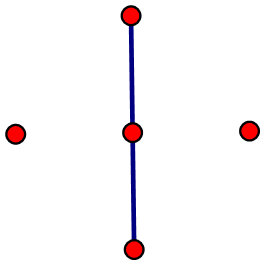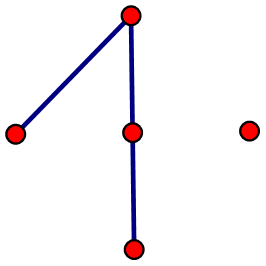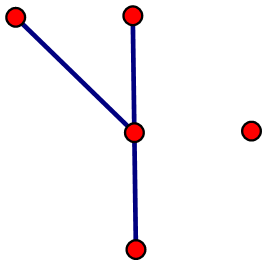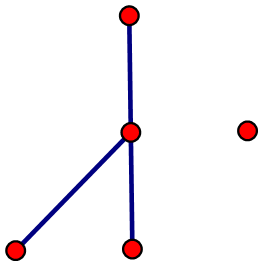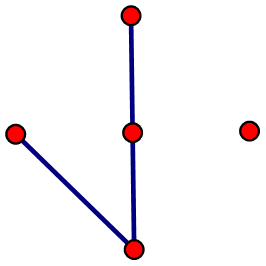2. 除最长链外，还有两条边的，共 7 种

11
12（最长反链只有 2）
13
14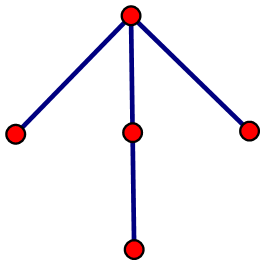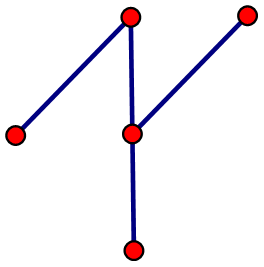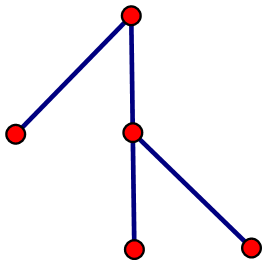21
22
23（最长反链只有 2）
24
同 12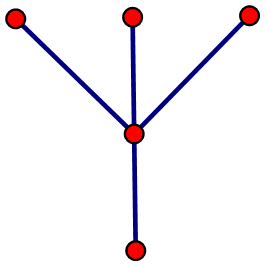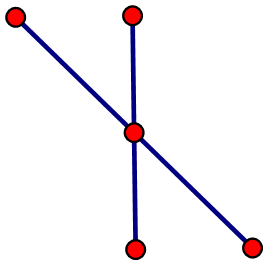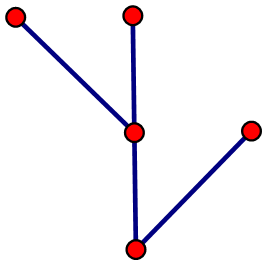31
32
33
34（最长反链只有 2）
同 13
同 23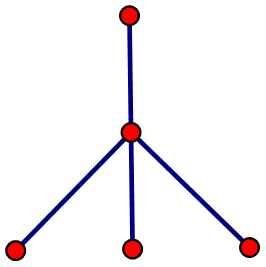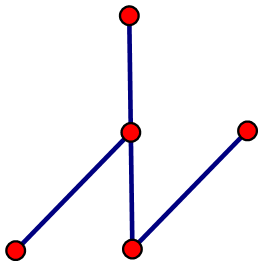41
42
43
44
同 14
同 24
同 34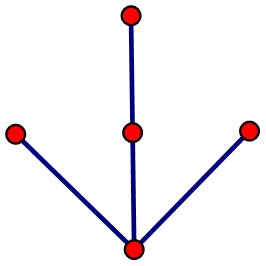3. 有多条最长链（有环）的，共 4 种

1
2
3
4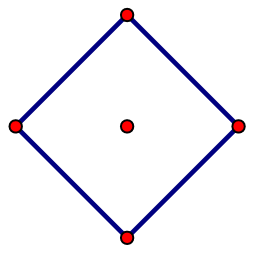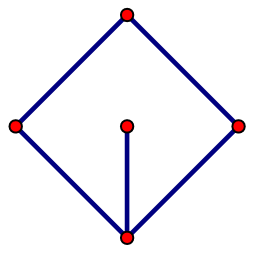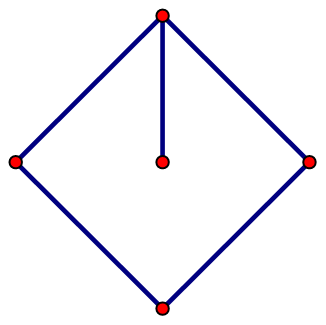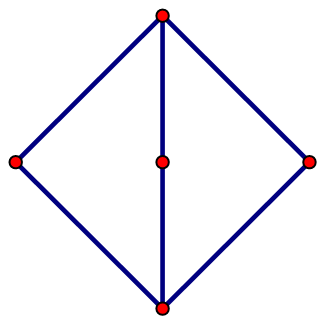### 第 32 题

$\oplus$ 表示对称差，求证：$\left(\bigcap\limits_{i=1}^n A_i\right)\oplus \left(\bigcap\limits_{i=1}^n B_i\right)=\bigcup\limits_{i=1}^n(A_i\oplus B_i)$.

1. $x$ 属于所有的 $A$，但至少不属于某一个 $B$. 不妨设 $x\notin B_i$，则 $x\in A_i\oplus B_i\subseteq \operatorname{RHS}$
2. $x$ 属于所有的 $B$，但至少不属于某一个 $A$. 不妨设 $x\notin A_j$，则 $x\in A_j\oplus B_j\subseteq \operatorname{RHS}$.

OK. 这样证明就结束了，简洁又直观.

1. $n=1$ 时，$\operatorname{LHS}=\operatorname{RHS}$

2. 设当 $n=k$ 时原式成立，则 $n=k+1$ 时（令 $A=\bigcap\limits_{i=1}^k A_i$$B=\bigcap\limits_{i=1}^k B_i$），有： \begin{align} \operatorname{LHS} &= (A\cap A_{n+1})\oplus (B\cap B_{n+1}) \\ &= \big((A\cap A_{n+1})\setminus (B\cap B_{n+1})\big) \cup \big( (B\cap B_{n+1})\setminus (A\cap A_{n+1}) \big) \\ &= \big((A\cap A_{n+1})\cap \overline{B\cap B_{n+1}}\big) \cup \big((B\cap B_{n+1})\cap \overline{A\cap A_{n+1}}\big) \\ &= \big((A\cap A_{n+1})\cap (\overline{B}\cup \overline{B_{n+1}})\big) \cup \big((B\cap B_{n+1})\cap (\overline{A}\cup \overline{A_{n+1}})\big) \\ &= (A\cap A_{n+1}\cap \overline{B})\cup (A\cap A_{n+1}\cap \overline{B_{n+1}}) \cup (B\cap B_{n+1}\cap \overline{A})\cup (B\cap B_{n+1}\cap \overline{A_{n+1}}) \\ &= (A_{n+1}\cap A\setminus B)\cup (A\cap A_{n+1}\setminus B_{n+1}) \cup (B_{n+1}\cap B\setminus A)\cup (B\cap B_{n+1}\setminus A_{n+1}) \\ &\subseteq (A\setminus B)\cup (A_{n+1}\setminus B_{n+1}) \cup (B\setminus A)\cup (B_{n+1}\setminus A_{n+1}) \\ &= (A\oplus B)\cup (A_{n+1}\oplus B_{n+1}) = \operatorname{RHS}. \end{align}

PART 2: Graph Theory

## 所有奇圈共点 $\Rightarrow$ 可被 5-染色

$\chi(G)\geqslant 6$，考虑颜色 $1,2,3$ 的导出子图 $G_1$ 和颜色 $4,5,6$ 的导出子图 $G_2$，则 $G_1\cap G_2=\varnothing$. 由于 $\chi(G_1)=\chi(G_2)=3$，故二者均不是二部图，因此 $G_1$$G_2$ 均含有奇圈. 又由于 $G_1$$G_2$ 无公共点，故这两个奇圈也无公共点，矛盾. 因此 $\chi(G)\leqslant 5$.